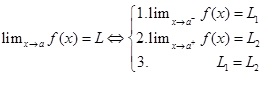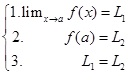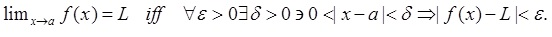Informal Definition of continuity:

f(x) is continuous on the interval [a,b] iff you can draw the function from a to b without lifting your pencil

Informal definition of limit:Formal Definition of Continuity:

f(x) is continuous on the interval [a, b] iffFormal Definition of Limit:Hopefully your browser will be able to detect all the symbols in this math text script. Here it is in English.

The limit as x approaches a of f(x) is L iff and only if for all epsilon greater than zero there exists a delta greater than zero such that the magnitude of the distance between x and a being less than delta will force the distance between f(x) and L to be less than epsilon.